### Home > PC > Chapter 4 > Lesson 4.2.4 > Problem4-112

4-112.
1. Verify the following trigonometric identities. Intermediate steps may involve complex fractions. Homework Help ✎

1. cot2 x(sec2 x − 1) = 1

2. sin2 α − cos2 α = 1 − 2 cos2 α

3. tan2 β + 6 = sec2 β + 5

4. cos y + sin y · tan y = sec y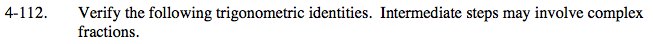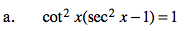Substitute a Pythagorean Identity for sec2x.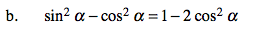Substitute the Fundamental Pythagorean Identity into the left side of the equation for sin2α.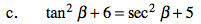Substitute a Pythagorean Identity for sec2x.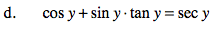Rewrite tan y as sin y / cos y.
Get common denominators and simplify.